# Dynamics of almost periodic solutions for a discrete Fox harvesting model with feedback control

## Abstract

We consider the following discrete Fox harvesting model with feedback control of the form

${ x ( n + 1 ) = x ( n ) exp { β ( n ) ln r ( K ( n ) x ( n ) ) − α ( n ) − γ ( n ) u ( n ) } , Δ u ( n ) = − μ ( n ) u ( n ) + ν ( n ) x ( n ) .$

Under the assumptions of almost periodicity of the coefficients, sufficient conditions are established for the existence and uniformly asymptotical stability of almost periodic solutions of this model. The persistence as well as the boundedness of solutions of the above system are discussed prior to presenting the main result. Examples are provided to illustrate the effectiveness of the proposed results.

MSC:39A11, 34K14.

## 1 Introduction

Consider the following equation of population dynamics [1, 2]:

$x ′ (t)=−xF(t,x)+xG(t,x), x ′ (t)= d x d t ,$
(1)

where $x=x(t)$ is the size of the population, $F(t,x)$ is the per-capita harvesting rate and $G(t,x)$ is the per-capita fecundity rate. Let $G(t,x)$ and $F(t,x)$ be defined in the form

$F(t,x)=α(t)andG(t,x)=β(t) ln r ( K ( t ) x ( t ) ) ,r>0,$

then equation (1) becomes

$x ′ (t)=x(t) [ β ( t ) ln r ( K ( t ) x ( t ) ) − α ( t ) ] ,$
(2)

where $α(t)$ is a variable harvesting rate, $β(t)$ is an intrinsic factor and $K(t)$ is a varying environmental carrying capacity. The positive parameter r is referred to as an interaction parameter [1, 3, 4]. Indeed, if $r>1$ then intra-specific competition is high, whereas if $0, then the competition is low. For $r=1$, equation (2) reduces to the classical Gompertzian model with harvesting [2, 5]. Equation (2) is called a Fox surplus production model that has been used to build up certain prediction models such as microbial growth model, demographic model and fisheries model. This equation is considered to be an efficient alternative to the well known r-logistic model. Specifically, the Fox model is more appropriate upon describing lower population density; we refer the reader to [1, 3, 4, 610] and, in particular, to the recent paper  for more information.

Ecosystems in the real world are continuously disturbed by unpredictable forces which can result in changing some biological parameters such as survival rates. In ecology, a question of practical interest is whether or not an ecosystem can withstand these unpredictable disturbances which persist for a finite period of time. In the language of control theory, we call these disturbance functions a control variable. In , Gopalsamy and Weng introduced a model with feedback controls in which the control variables satisfy a certain differential equation. The next years have witnessed the appearance of many papers regarding the study of ecosystems with feedback control; see for instance .

In the last years, many authors have argued that the discrete time models governed by difference equations are more appropriate than the continuous counterparts, especially when the populations have no overlapping generations. It is also known that the discrete models can provide more efficient computational methods for numerical simulations . By applying the same method used in , one can derive the discrete analogue of (2) as follows:

$x(n+1)=x(n)exp { β ( n ) ln r ( K ( n ) x ( n ) ) − α ( n ) } .$
(3)

One of the most important behaviors of solutions which has been the main object of investigations among authors is the periodic behavior of solutions . To consider periodic environmental factors acting on a population model, it is natural to study the model subject to periodic coefficients. Indeed, the assumption of periodicity of the parameters in the model is a way of incorporating the time-dependent variability of the environment (e.g., seasonal effects of weather, food supplies, mating habits and harvesting). On the other hand, upon considering long-term dynamical behavior, it has been found that the periodic parameters often turn out to experience some perturbations that may lead to a change in character. Thus, the investigation of almost periodic behavior is considered to be in more accordance with reality; see the remarkable monographs  and the recent contributions .

Motivated by the above justifications, we consider the following discrete Fox harvesting model with feedback control in the form

${ x ( n + 1 ) = x ( n ) exp { β ( n ) ln r ( K ( n ) x ( n ) ) − α ( n ) − γ ( n ) u ( n ) } , Δ u ( n ) = − μ ( n ) u ( n ) + ν ( n ) x ( n ) ,$
(4)

where $u(n)$ is the control variable and $Δu(n)$ is the forward difference $u(n+1)−u(n)$. Under the assumptions of almost periodicity of coefficients of system (4), we shall study the existence and uniformly asymptotical stability of almost periodic solutions for system (4). The persistence as well as the boundedness of solutions of system (4) are discussed prior to presenting the main result. To the best of author’s observation, no paper has been published in the literature regarding the dynamics of almost periodic solutions of system (4). Thus, the result of this paper is essentially different and presents a new approach.

The remaining part of this paper is organized as follows. In Section 2, some preliminary definitions along with essential lemmas are given. Section 9 discusses the persistence and boundedness of solutions of system (4). In Section 30, sufficient conditions are established to investigate the existence and uniformly asymptotical stability of almost periodic solutions of the said system. Section 5 provides some numerical examples to illustrate the feasibility of our theoretical results.

## 2 Preliminaries

Let $R$, $R +$, $Z$ and $Z +$ be the sets of real, nonnegative real, integer and nonnegative integer numbers respectively. For any bounded sequence ${f(n)}$ on $Z$, we define

$f M = sup n ∈ Z f(n)and f m = inf n ∈ Z f(n).$
(5)

Throughout the remainder of this paper, we assume the following condition:

(H.1) ${β(n)}$, ${K(n)}$, ${α(n)}$, ${γ(n)}$, ${μ(n)}$ and ${ν(n)}$ are bounded nonnegative almost periodic sequences such that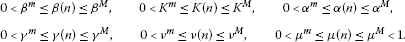Due to certain biological reasons, we restrict our attention to positive solutions of system (4). Thus, we consider system (4) together with the following initial conditions:

$x(0)>0andu(0)>0.$
(6)

One can easily figure out that the solutions of system (4) with the initial conditions (6) are defined and remain positive for all $n∈ Z +$.

Definition 1 

A sequence $x:Z→R$ is called an almost periodic sequence if the ϵ-translation set $E{ϵ,x}={τ∈Z:|x(n+τ)−x(n)<ϵ,∀n∈Z}$ is a relatively dense set in $Z$ for all $ϵ>0$, that is, for any $ϵ>0$ there is a constant $l(ϵ)>0$ such that in any interval of length $l(ϵ)$ there exists a number $τ∈E{ϵ,x}$ such that the inequality

$|x(n+τ)−x(n)|<ϵ$

is satisfied for all $n∈Z$.

Definition 2 

Let $f:Z×D→R$ where D is an open set in $R$. Then $f(n,x)$ is said to be almost periodic in n uniformly for $x∈D$, or uniformly almost periodic for short, if for any $ϵ>0$ and any compact set $S∈D$, there exists a positive integer $l(ϵ,S)$ such that any interval of length $l(ϵ,S)$ contains an integer τ for which

$|f(n+τ,x)−f(n,x)|<ϵ$

for all $n∈Z$ and $x∈S$. The number τ is called the ϵ-translation for $f(n,x)$.

Lemma 1 

${x(n)}$ is an almost periodic sequence if and only if for any sequence ${ h k ′ }⊂Z$, there exists a subsequence ${ h k }⊂{ h k ′ }$ such that $x(n+ h k )$ converges uniformly on $n∈Z$ as $k→∞$. Furthermore, the limit sequence is also an almost periodic sequence.

Consider the following almost periodic difference system:

$x(n+1)=f ( n , x ( n ) ) ,n∈ Z + ,$
(7)

where $f:Z× S δ →R$, $S δ ={x∈R:∥x∥<δ}$ and $f(n,x)$ is almost periodic in n uniformly for $x∈ S δ$ and is continuous in x. The product system of (7) is in the form

$x(n+1)=f ( n , x ( n ) ) ,y(n+1)=f ( n , y ( n ) ) .$
(8)

Our approach is based on the following lemma.

Lemma 2 

Suppose that there exists a Lyapunov functional $V(n,x,y)$ defined for $n∈ Z +$, $∥x∥<δ$, $∥y∥<δ$ and satisfying the following conditions:

1. (i)

$a(∥x−y∥)≤V(n,x,y)≤b(∥x−y∥)$, where $a,b∈T$ with $T={a∈C( R + , R + ):a(0)=0 and a is increasing }$;

2. (ii)

$|V(n, x 1 , y 1 )−V(n, x 2 , y 2 )|≤L(∥ x 1 − x 2 ∥+∥ y 1 − y 2 ∥)$ where $L>0$ is a constant;

3. (iii)

$Δ ( 8 ) V(n,x,y)≤−λV(n,x,y)$ where $0<λ<1$ is a constant and $Δ ( 8 ) V(n,x,y)=V(n+1,f(n,x),f(n,y))−V(n,x,y)$.

Moreover, if there exists a solution $φ(n)$ of system (8) such that $∥φ(n)∥≤ δ ∗ <δ$ for $n∈ Z +$, then there exists a unique uniformly asymptotically stable almost periodic solution $p(n)$ of system (8) which satisfies $∥p(n)∥≤ δ ∗$. In particular, if $f(n,x)$ is periodic of period ω, then there exists a unique uniformly asymptotically stable periodic solution of system (8) of period ω.

## 3 Persistence and boundedness

In this section, we prove every solution of system (4) is persistent. In addition to this, we prove that there exists a bounded solution for (4).

Definition 3 System (4) is said to be persistent if there are positive constants $x ∗$, $u ∗$, $x ∗$ and $u ∗$ such that

$lim sup n → ∞ x(n)≤ x ∗ , lim sup n → ∞ u(n)≤ u ∗$

and

$lim inf n → ∞ x(n)≥ x ∗ , lim sup n → ∞ u(n)≥ u ∗$

for each positive solution $(x(n),u(n))$ of (4).

We assume the following condition:

(H.2) $β m ln r K m > α M + γ M u ∗$.

Set

$x ∗ = K M e [ ( α β ) m ] − 1 r exp { ( β ln r K ) M } and u ∗ = ν M x ∗ μ m .$

Lemma 3 Let (H.1), (H.2) hold. Then, every solution of system (4) satisfies

$lim sup n → ∞ x(n)≤ x ∗ and lim sup n → ∞ u(n)≤ u ∗ .$

Proof Let $(x(n),u(n))$ be a solution of (4). To prove that $lim sup n → ∞ x(n)≤ x ∗$, we consider two cases:

Case I. There exists $l 0 ∈ Z +$ such that $x( l 0 +1)≥x( l 0 )$. By the first equation of (4), we have

$β( l 0 ) ln r ( K ( l 0 ) x ( l 0 ) ) −α( l 0 )−γ( l 0 )u( l 0 )≥0,$

which implies that

$x( l 0 )≤ K M e [ ( α β ) m ] − 1 r ≤ x ∗ .$

Thus,

$x ( l 0 + 1 ) = x ( l 0 ) exp { β ( l 0 ) ln r ( K ( l 0 ) x ( l 0 ) ) − α ( l 0 ) − γ ( l 0 ) u ( l 0 ) } ≤ K M e [ ( α β ) m ] − 1 r exp { ( β ln r K ) M } = x ∗ .$

We claim that $x(n)≤ x ∗$ for $n≥ l 0$. Indeed, if there is an integer $n 0 ≥ l 0 +2$ such that $x( n 0 )> x ∗$ and $n 1$ is the least integer between $l 0$ and $n 0$ such that $x( n 1 )= max l 0 ≤ n ≤ n 0 {x(n)}$, then $n 1 ≥ l 0 +2$ and $x( n 1 )>x( n 1 −1)$ which implies that $x( n 1 )≤ x ∗ . This is a contradiction. This proves the claim.

Case II. Let $x(n)≥x(n+1)$ for $n∈ Z +$. Then, $lim n → ∞ x(n)$ exists and equals $x ¯$. Taking the limit of the first equation in (4), we have

$lim n → ∞ ( β ( n ) ln r ( K ( n ) x ( n ) ) − α ( n ) − γ ( n ) u ( n ) ) =0.$

Hence $x ¯ ≤ K M e [ ( α β ) m ] − 1 r ≤ x ∗$. This proves the claim.

Now, we prove that $lim sup n → ∞ u(n)≤ u ∗$. For any $ε>0$, there exists a large enough integer $n 0 ∈ Z +$ such that $x(n)≤ x ∗ +ε$ for $n≥ n 0$. By the second equation of (4), we get

$u ( n ) = ∏ i = 0 n − 1 ( 1 − μ ( i ) ) [ u ( 0 ) + ∑ i = 0 n − 1 ν ( i ) x ( i ) ∏ j = 0 i ( 1 − μ ( j ) ) ] ≤ ( 1 − μ m ) n [ u ( 0 ) + ∑ i = 0 n 0 − 1 ν ( i ) x ( i ) ∏ j = 0 i ( 1 − μ ( j ) ) ] + ν M ( x ∗ + ε ) ∑ i = n 0 n − 1 ∏ j = i − 1 n − 1 ( 1 − μ ( j ) ) ≤ ( 1 − μ m ) n [ u ( 0 ) + ∑ i = 0 n 0 − 1 ν ( i ) x ( i ) ∏ j = 0 i ( 1 − μ ( j ) ) ] + ν M ( x ∗ + ε ) ∑ i = n 0 n − 1 ( 1 − μ m ) n − i − 1 .$

Since $0< μ m <1$, we can find a positive number d such that $1− μ m = e − d$. Thus, by using Stolz’s theorem, we obtain

$lim n → ∞ ∑ i = n 0 n − 1 ( 1 − μ m ) n − i − 1 = lim n → ∞ ∑ i − n 0 n − 1 e d ( i + 1 ) e d n = 1 1 − e − d = 1 μ m .$

Hence

$lim sup n → ∞ u(n)≤ ν M ( x ∗ + ε ) μ m .$

By the arbitrariness of ε, we obtain $lim sup n → ∞ u(n)≤ ν M x ∗ μ m := u ∗$. The proof of Lemma 3 is complete. □

Set

$x ∗ = K m e ( α M + γ M ( u ∗ ) β m ) − 1 r exp { β m ln r ( K m x ∗ ) − α M − γ M u ∗ } and u ∗ = ν m x ∗ μ M .$

Lemma 4 Let (H.1), (H.2) hold. Then every solution of system (4) satisfies

$lim inf n → ∞ x(n)≥ x ∗ and lim inf n → ∞ u(n)≥ u ∗ .$

Proof Let $(x(n),u(n))$ be a solution of (4). By virtue of Lemma 3, one can figure out that for any $ε>0$ which satisfies $β m ln r K m > α M + γ M ( u ∗ +ε)$, there exists $n 0 ∈ Z +$ such that

To prove that $lim inf n → ∞ x(n)≥ x ∗$, we consider two cases:

Case I. There exists $l 0 ≥ n 0$ such that $x( l 0 +1)≤x( l 0 )$. We observe that for $n≥ l 0$, we have

$x ( n + 1 ) = x ( n ) exp { β ( n ) ln r ( K ( n ) x ( n ) ) − α ( n ) − γ ( n ) u ( n ) } ≥ x ( n ) exp { β m ln r ( K ( n ) x ( n ) ) − α M − γ M ( u ∗ + ε ) } .$

For $n= l 0$, we get

$β m ln r ( K ( l 0 ) x ( l 0 ) ) − α M − γ M ( u ∗ + ε ) ≤0,$

which implies that

$x( l 0 )≥ K m e ( α M + γ M ( u ∗ + ε ) β m ) − 1 r .$

It follows that

$x ( l 0 + 1 ) = x ( l 0 ) exp { β ( l 0 ) ln r ( K ( l 0 ) x ( l 0 ) ) − α ( l 0 ) − γ ( l 0 ) u ( l 0 ) } ≥ K m e ( α M + γ M ( u ∗ + ε ) β m ) − 1 r exp { β m ln r ( K m x ∗ + ε ) − α M − γ M ( u ∗ + ε ) } .$

Let

$x ε = K m e ( α M + γ M ( u ∗ + ε ) β m ) − 1 r exp { β m ln r ( K m x ∗ + ε ) − α M − γ M ( u ∗ + ε ) } .$

We claim that

For the sake of contradiction, assume that there exists $p 0 ≥ l 0$ such that $x( p 0 )< x ε$. Then $p 0 ≥ l 0 +2$. Let $p 1 ≥ l 0 +2$ be the smallest integer such that $x( p 1 )< x ε$. Then $x( p 1 −1)>x( p 1 )$. The above arguments imply that $x( p 1 )≥ x ε$ which is a contradiction. This proves the claim.

Case II. Let $x(n)>x(n+1)$ for all $n∈ Z +$. Then, $lim n → ∞ x(n)$ exists and it is equal to $x ̲$. Taking the limit of the first equation of (4), we have

$lim n → ∞ ( β ( n ) ln r ( K ( n ) x ( n ) ) − α ( n ) − γ ( n ) u ( n ) ) =0.$

Hence $x ̲ ≥ K m e ( α M + γ M ( u ∗ + ε ) β m ) − 1 r ≥ x ε$ and $lim ε → 0 x ε = x ∗$. This proves the claim.

By applying the same arguments followed in the proof of Lemma 3, one can easily show that $lim n → ∞ u(n)≥ u ∗$. The proof of Lemma 4 is complete. □

The results of Lemma 3 and Lemma 4 can be concluded in the following theorem:

Theorem 1 Let (H.1), (H.2) hold. Then system (4) is persistent.

Let Ω be the set of all solutions $(x(n),u(n))$ of system (4) satisfying $x ∗ ≤x(n)≤ x ∗$ and $u ∗ ≤u(n)≤ u ∗$ for all $n∈ Z +$. By virtue of Theorem 1, it should be noted that Ω is an invariant set of system (4).

In view of Lemma 2, we need to show that there exists a bounded solution of system (4). The following result proves the existence of such a solution.

Theorem 2 Let (H.1), (H.2) hold. Then $Ω≠∅$.

Proof By the almost periodicity of ${β(n)}$, ${K(n)}$, ${α(n)}$, ${γ(n)}$, ${μ(n)}$ and ${ν(n)}$, there exists an integer valued sequence ${ τ p }$ with ${ τ p }→∞$ as $p→∞$ such that $β(n+ τ p )→β(n)$, $K(n+ τ p )→K(n)$, $α(n+ τ p )→α(n)$, $γ(n+ τ p )→γ(n)$, $μ(n+ τ p )→μ(n)$, $ν(n+ τ p )→ν(n)$ as $p→∞$. Let ε be an arbitrary small positive number. It follows from Lemma 3 and Lemma 4 that there exists a positive integer $N 0$ such that

Let $x p (n)=x(n+ τ p )$ and $u p (n)=u(n+ τ p )$ for $n≥ N 0 − τ p$, $p=1,2,…$ . For any positive integer q, it is easy to see that there exist sequences ${ x p (n):p≥q}$ and ${ u p (n):p≥q}$ such that the sequences ${ x p (n)}$ and ${ u p (n)}$ have subsequences, denoted by ${ x p (n)}$ and ${ u p (n)}$ again, converging on any finite interval of $Z$ as $p→∞$, respectively. Thus, we have sequences ${z(n)}$ and ${w(n)}$ such that

Therefore, the system

${ x p ( n + 1 ) = x p ( n ) exp { β ( n + τ p ) ln r ( K ( n + τ p ) x p ( n ) ) − α ( n + τ p ) − γ ( n + τ p ) u p ( n ) } , u p ( n + 1 ) = ( 1 − μ ( n + τ p ) ) u p ( n ) + ν ( n + τ p ) x p ( n ) ,$
(9)

implies

${ z ( n + 1 ) = z ( n ) exp { β ( n ) ln r ( K ( n ) z ( n ) ) − α ( n ) − γ ( n ) w ( n ) } , w ( n ) = ( 1 − μ ( n ) ) w ( n ) + ν ( n ) z ( n ) .$
(10)

We can easily see that $(z(n),w(n))$ is a solution of system (4) and $x ∗ −ε≤z(n)≤ x ∗ +ε$, $u ∗ −ε≤w(n)≤ u ∗ +ε$ for $n∈Z$. Since ε is arbitrary, it follows that $x ∗ ≤z(n)≤ x ∗$, $u ∗ ≤w(n)≤ u ∗$ for $n∈Z$. This completes the proof. □

## 4 The main result

Let $f(x)= ln r ( K ( n ) e x )$, where $x∈[ln x ∗ ,ln x ∗ ]$. Then, it is easy to find out that $f ′ (x)=−r [ ln ( K ( n ) e x ) ] r − 1$. The following inequalities hold:

$−r [ ln ( K ( n ) e x ) ] r − 1 ≤−r [ ln ( K m x ∗ ) ] r − 1 := Φ 1$
(11)

and

$r [ ln ( K ( n ) e x ) ] r − 1 ≤r ln r − 1 K M := Φ 2 .$
(12)

Theorem 3 Let (H.1), (H.2) hold. Suppose further that

(H.3) $0<Γ<1$ for $Γ=min{ Γ 1 , Γ 2 }$, where

$Γ 1 =− β M 2 Φ 2 2 − γ M − γ M β M Φ 2 −2 β M Φ 1 − ν M 2 x ∗ 2 + ν M x ∗ ( μ m − 1 )$

and

$Γ 2 =− γ M 2 − γ M β M Φ 2 − γ M + μ m (2− μ M )− ν M x ∗ ( 1 − μ m ) .$

Then, there exists a unique uniformly asymptotically stable almost periodic solution $X=(x(n),u(n))$ of system (4) which satisfies $x ∗ ≤x(n)≤ x ∗$ and $u ∗ ≤u(n)≤ u ∗$ for all $n∈ Z +$.

Proof Let $p(n)=lnx(n)$. In view of system (4), we have

${ p ( n + 1 ) = p ( n ) + β ( n ) ln r ( K ( n ) x ( n ) ) − α ( n ) − γ ( n ) u ( n ) , Δ u ( n ) = − μ ( n ) u ( n ) + ν ( n ) e p ( n ) .$
(13)

By the result of Theorem 2, it follows that system (13) has a bounded solution $(p(n),u(n))$ satisfying

Hence, $|p(n)|≤σ$ and $|u(n)|≤ρ$, where $σ=max{|ln x ∗ |,|ln x ∗ |}$ and $ρ=max{ u ∗ , u ∗ }$.

For $(p,u)∈ R 2$, we define the norm $∥(p,u)∥=|p|+|u|$. Suppose that $X=(p(n),u(n))$ and $Y=(q(n),v(n))$ are any two solutions of system (13) defined on $Z + × Ω ∗ × Ω ∗$, then $∥X∥≤κ$ and $∥Y∥≤κ$ where $κ=σ+ρ$ and .

Consider the product system of (13)

${ p ( n + 1 ) = p ( n ) + β ( n ) ln r ( K ( n ) e p ( n ) ) − α ( n ) − γ ( n ) u ( n ) , Δ u ( n ) = − μ ( n ) u ( n ) + ν ( n ) e p ( n ) , q ( n + 1 ) = q ( n ) + β ( n ) ln r ( K ( n ) e q ( n ) ) − α ( n ) − γ ( n ) v ( n ) , Δ v ( n ) = − μ ( n ) v ( n ) + ν ( n ) e q ( n ) .$
(14)

Construct a Lyapunov function defined on $Z + × Ω ∗ × Ω ∗$ as follows:

$V(n,X,Y)= ( p ( n ) − q ( n ) ) 2 + ( u ( n ) − v ( n ) ) 2 .$

It is easy to see that the norm $∥X−Y∥=|p(n)−q(n)|+|u(n)−v(n)|$ and the norm $∥ X − Y ∥ ∗ = { ( p ( n ) − q ( n ) ) 2 + ( u ( n ) − v ( n ) ) 2 } 1 2$ are equivalent, that is, there exist two constants $C 1 >0$ and $C 2 >0$ such that

$C 1 ∥X−Y∥≤ ∥ X − Y ∥ ∗ ≤ C 2 ∥X−Y∥.$

Thus

$( C 1 ∥ X − Y ∥ ) 2 ≤ ∥ X − Y ∥ ∗ 2 ≤ ( C 2 ∥ X − Y ∥ ) 2$

or

$( C 1 ∥ X − Y ∥ ) 2 ≤V(n,X,Y)≤ ( C 2 ∥ X − Y ∥ ) 2 .$

Let $a,b∈C( R + , R + )$ such that $a(x)= C 1 2 x 2$ and $b(x)= C 2 2 x 2$. Thus, condition (i) of Lemma 2 is satisfied.

Moreover,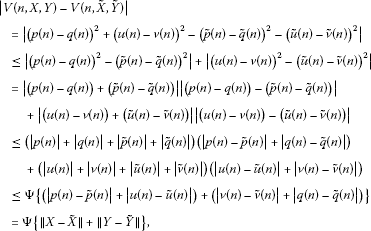where $X ˜ =( p ˜ (n), u ˜ (n))$, $Y ˜ =( q ˜ (n), v ˜ (n))$ and $Ψ=4max{σ,ρ}$. Therefore, condition (ii) of Lemma 2 is satisfied.

Finally, we calculate the difference $ΔV(n,X,Y)$ along system (14). Indeed,

$Δ V ( 14 ) ( n , X , Y ) = V ( n + 1 , X , Y ) − V ( n , X , Y ) = ( p ( n + 1 ) − q ( n + 1 ) ) 2 − ( p ( n ) − q ( n ) ) 2 + ( u ( n + 1 ) − v ( n + 1 ) ) 2 − ( u ( n ) − v ( n ) ) 2 .$
(15)

In view of system (15), we observe that

$( p ( n + 1 ) − q ( n + 1 ) ) 2 = [ ( p ( n ) − q ( n ) ) + β ( n ) ln r ( K ( n ) e p ( n ) ) − β ( n ) ln r ( K ( n ) e q ( n ) ) − γ ( n ) ( u ( n ) − v ( n ) ) ] 2$

or

$( p ( n + 1 ) − q ( n + 1 ) ) 2 = ( p ( n ) − q ( n ) ) 2 + [ β ( n ) ln r ( K ( n ) e p ( n ) ) − β ( n ) ln r ( K ( n ) e q ( n ) ) − γ ( n ) ( u ( n ) − v ( n ) ) ] 2 + 2 ( p ( n ) − q ( n ) ) [ β ( n ) ln r ( K ( n ) e p ( n ) ) − β ( n ) ln r ( K ( n ) e q ( n ) ) − γ ( n ) ( u ( n ) − v ( n ) ) ] .$

Thus,

$( p ( n + 1 ) − q ( n + 1 ) ) 2 = ( p ( n ) − q ( n ) ) 2 + [ β ( n ) ln r ( K ( n ) e p ( n ) ) − β ( n ) ln r ( K ( n ) e q ( n ) ) ] 2 + γ 2 ( n ) ( u ( n ) − v ( n ) ) 2 − 2 γ ( n ) ( u ( n ) − v ( n ) ) [ β ( n ) ln r ( K ( n ) e p ( n ) ) − β ( n ) ln r ( K ( n ) e q ( n ) ) ] + 2 ( p ( n ) − q ( n ) ) [ β ( n ) ln r ( K ( n ) e p ( n ) ) − β ( n ) ln r ( K ( n ) e q ( n ) ) ] − 2 γ ( n ) ( p ( n ) − q ( n ) ) ( u ( n ) − v ( n ) ) .$
(16)

Moreover,

$( u ( n + 1 ) − v ( n + 1 ) ) 2 = [ ( 1 − μ ( n ) ) u ( n ) + ν ( n ) e p ( n ) − ( 1 − μ ( n ) ) v ( n ) − ν ( n ) e q ( n ) ] 2 = [ ( 1 − μ ( n ) ) ( u ( n ) − v ( n ) ) + ν ( n ) ( e p ( n ) − e q ( n ) ) ] 2$

or

$( u ( n + 1 ) − v ( n + 1 ) ) 2 = ( u ( n ) − v ( n ) ) 2 + μ ( n ) ( μ ( n ) − 2 ) ( u ( n ) − v ( n ) ) 2 + ν 2 ( n ) ( e p ( n ) − e q ( n ) ) 2 + 2 ν ( n ) ( 1 − μ ( n ) ) ( u ( n ) − v ( n ) ) ( e p ( n ) − e q ( n ) ) .$
(17)

Substituting (16) and (17) back in (15), we obtain

$Δ V ( n , X , Y ) = [ β ( n ) ln r ( K ( n ) e p ( n ) ) − β ( n ) ln r ( K ( n ) e q ( n ) ) ] 2 + γ 2 ( n ) ( u ( n ) − v ( n ) ) 2 − 2 γ ( n ) ( u ( n ) − v ( n ) ) [ β ( n ) ln r ( K ( n ) e p ( n ) ) − β ( n ) ln r ( K ( n ) e q ( n ) ) ] + 2 ( p ( n ) − q ( n ) ) [ β ( n ) ln r ( K ( n ) e p ( n ) ) − β ( n ) ln r ( K ( n ) e q ( n ) ) ] − 2 γ ( n ) ( p ( n ) − q ( n ) ) ( u ( n ) − v ( n ) ) + μ ( n ) ( μ ( n ) − 2 ) ( u ( n ) − v ( n ) ) 2 + ν 2 ( n ) ( e p ( n ) − e q ( n ) ) 2 + 2 ν ( n ) ( 1 − μ ( n ) ) ( u ( n ) − v ( n ) ) ( e p ( n ) − e q ( n ) ) .$
(18)

By applying the Mean Value Theorem, we have

$ln r ( K ( n ) e p ( n ) ) − ln r ( K ( n ) e q ( n ) ) =−r [ ln ( K ( n ) e ξ ( n ) ) ] r − 1 ( p ( n ) − q ( n ) )$
(19)

and

$e p ( n ) − e q ( n ) = e η ( n ) ( p ( n ) − q ( n ) ) ,$
(20)

where $ξ(n)$, $η(n)$ lie between $p(n)$ and $q(n)$. Substituting (19) and (20) back in (18), we get

$ΔV(n,X,Y)= Ω 1 + Ω 2 + Ω 3 + Ω 4 + Ω 5 + Ω 6 + Ω 7 + Ω 8 ,$
(21)

where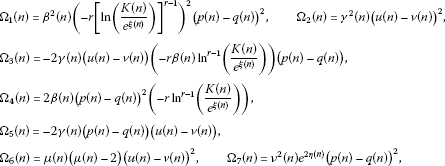and

$Ω 8 (n)=2ν(n) e η ( n ) ( 1 − μ ( n ) ) ( u ( n ) − v ( n ) ) ( p ( n ) − q ( n ) ) .$

By virtue of (H.1), (11) and (12), we observe that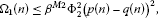(22)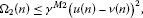(23)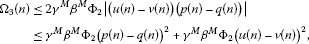(24)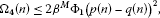(25)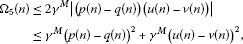(26)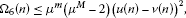(27)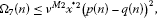(28)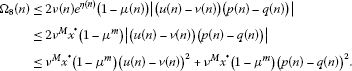(29)

Substituting (22)-(29) back in (21), we obtain

$Δ V ( n , X , Y ) ≤ β M 2 Φ 2 2 ( p ( n ) − q ( n ) ) 2 + γ M 2 ( u ( n ) − v ( n ) ) 2 + γ M β M Φ 2 ( p ( n ) − q ( n ) ) 2 + γ M β M Φ 2 ( u ( n ) − v ( n ) ) 2 + 2 β M Φ 1 ( p ( n ) − q ( n ) ) 2 + γ M ( p ( n ) − q ( n ) ) 2 + γ M ( u ( n ) − v ( n ) ) 2 + μ m ( μ M − 2 ) ( u ( n ) − v ( n ) ) 2 + ν M 2 x ∗ 2 ( p ( n ) − q ( n ) ) 2 + ν M x ∗ ( 1 − μ m ) ( u ( n ) − v ( n ) ) 2 + ν M x ∗ ( 1 − μ m ) ( p ( n ) − q ( n ) ) 2 = − [ − β M 2 Φ 2 2 − γ M − γ M β M Φ 2 − 2 β M Φ 1 − ν M 2 x ∗ 2 + ν M x ∗ ( μ m − 1 ) ] ( p ( n ) − q ( n ) ) 2 − [ − γ M 2 − γ M β M Φ 2 − γ M + μ m ( 2 − μ M ) − ν M x ∗ ( 1 − μ m ) ] ( u ( n ) − v ( n ) ) 2 = − Γ { ( p ( n ) − q ( n ) ) 2 + ( u ( n ) − v ( n ) ) 2 } = − Γ V ( n , X , Y ) .$

By virtue of the condition that $0<Γ<1$, assumption (iii) of Lemma 2 is satisfied. Thus, we conclude that there exists a unique uniformly asymptotically stable almost periodic solution $X=(p(n),u(n))$ of system (13) which satisfies $ln x ∗ ≤p(n)≤ln x ∗$ and $u ∗ ≤u(n)≤ u ∗$ for all $n∈ Z +$. It follows that there exists a unique uniformly asymptotically stable almost periodic solution $X=(x(n),u(n))$ of system (4) which satisfies $x ∗ ≤x(n)≤ x ∗$ and $u ∗ ≤u(n)≤ u ∗$ for all $n∈ Z +$. □

Assume the following condition:

(H.4) ${β(n)}$, ${K(n)}$, ${α(n)}$, ${γ(n)}$, ${μ(n)}$ and ${ν(n)}$ are bounded nonnegative periodic sequences of period ω.

Corollary 1 Let (H.2)-(H.4) hold. Then system (4) has a unique uniformly asymptotically stable periodic solution of period ω.

## 5 Some examples

Example 1 Consider the following system:

${ x ( n + 1 ) = x ( n ) exp { ( 0.25 − 0.05 cos n ) ln 1 3 ( 1 x ( n ) ) − 0.2 − 0.2 u ( n ) } , Δ u ( n ) = − ( 0.35 − 0.05 sin π n ) u ( n ) + ( 0.4 ) x ( n ) ,$
(30)

where $β(n)=0.25−0.05cosn$, $α(n)=0.2$, $γ(n)=0.2$, $μ(n)=0.35−0.05sinπn$, $ν(n)=0.4$, $K(n)≡1$, $r= 1 3$. By calculation, we find $0.044≈ x ∗ < x ∗ ≈0.0498$, $0.044≈ u ∗ < u ∗ ≈0.0664$ and $Γ 1 ≈0.8166$, $Γ 2 ≈0.2260$. Therefore, $Γ≈0.2260$. One can easily check the validity of conditions (H.1)-(H.3). Thus, by Theorem 1 and Theorem 3, system (30) is persistent and has a unique uniformly asymptotically stable almost periodic solution.

Example 2 Consider the following system:

${ x ( n + 1 ) = x ( n ) exp { ( 0.35 − 0.05 sin 3 n ) ln ( 1 + 0.01 sin 3 n x ( n ) ) x ( n + 1 ) = − ( 0.2 + 0.01 sin 3 n ) − ( 0.0015 + 0.0005 sin 3 n ) u ( n ) } , Δ u ( n ) = − ( 0.35 − 0.05 cos 3 n ) u ( n ) + ( 0.0015 + 0.0005 sin 3 n ) x ( n ) ,$
(31)

where $β(n)=0.35−0.05sin 3 n$, $α(n)=0.2+0.01sin 3 n$, $γ(n)=0.0015+0.0005×sin 3 n$, $μ(n)=0.35−0.05cos 3 n$, $ν(n)=0.0015+0.0005sin 3 n$, $K(n)=1+0.01sin 3 n$, $r=2$. By calculation, we find $0.05823≈ x ∗ < x ∗ ≈0.73588$, $0.00145≈ u ∗ < u ∗ ≈0.0049$ and $Γ 1 ≈0.267226$, $Γ 2 ≈0.22317$. Therefore, $Γ≈0.22317$. One can easily check the validity of conditions (H.1)-(H.3). Thus, by Theorem 1 and Theorem 3, system (31) is persistent and has a unique uniformly asymptotically stable almost periodic solution.

Example 3 Consider the following system:

${ x ( n + 1 ) = x ( n ) exp { ( 0.25 − 0.05 sin n ) ln ( 1 x ( n ) ) x ( n + 1 ) = − ( 0.35 + 0.05 sin π n ) − ( 0.001 ) u ( n ) } , Δ u ( n ) = − ( 0.25 − 0.05 cos n ) u ( n ) + ( 0.02 ) x ( n ) ,$
(32)

where $β(n)=0.25−0.05sinn$, $α(n)=0.35+0.05sinπn$, $γ(n)=0.001$, $μ(n)=0.25−0.05cosn$, $ν(n)=0.02$, $K(n)≡1$, $r≡1$. By calculation, we find $0.12246≈ x ∗ < x ∗ ≈0.223$, $0.008164≈ u ∗ < u ∗ ≈0.0223$ and $Γ 1 ≈0.596$, $Γ 2 ≈0.335$. Therefore, $Γ≈0.335$. One can easily check the validity of conditions (H.2)-(H.4). Thus, by Theorem 1 and Corollary 1, system (32) is persistent and has a unique uniformly asymptotically stable periodic solution.

## References

1. 1.

Brauer F, Castillo-Chavez C: Mathematical Models in Population Biology and Epidemiology. Springer, Berlin; 2001.

2. 2.

Edelstein-Keshet L Classics in Applied Mathematics 46. In Mathematical Models in Biology. SIAM, Philadelphia; 2004.

3. 3.

Jensen A: Harvest in a fluctuating environment and conservative harvest for the Fox surplus production model. Ecol. Model. 2005, 182: 1–9. 10.1016/j.ecolmodel.2003.08.004

4. 4.

Tsoularis A, Wallace J: Analysis of logistic growth models. Math. Biosci. 2002, 179(1):21–55. 10.1016/S0025-5564(02)00096-2

5. 5.

Brauer F: Periodic environment and periodic harvesting. Nat. Resour. Model. 2003, 16: 233–244.

6. 6.

Fox W: An exponential surplus-yield model for optimizing exploited fish populations. Trans. Am. Fish. Soc. 1970, 99: 80–88. 10.1577/1548-8659(1970)99<80:AESMFO>2.0.CO;2

7. 7.

Kozusko F, Bajzer Z: Combining Gompartzian growth and cell population dynamics. Math. Biosci. 2003, 185(2):153–167. 10.1016/S0025-5564(03)00094-4

8. 8.

Cushing JM, Costantino RF, Dennis B, Desharnais RA, Henson SM: Nonlinear population dynamics: models, experiments and data. J. Theor. Biol. 1998, 194: 1–9. 10.1006/jtbi.1998.0736

9. 9.

Berezanskya L, Idels L: Periodic Fox production harvesting models with delay. Appl. Math. Comput. 2008, 195(1):142–153. 10.1016/j.amc.2007.04.084

10. 10.

Idels L: Stability analysis of periodic Fox production model. Can. Appl. Math. Q. 2006, 14(3):331–341.

11. 11.

Alzabut JO, Obaidat S: Almost periodic solutions for Fox production harvesting model with delay. Electron. J. Qual. Theory Differ. Equ. 2012, 34: 1–12.

12. 12.

Gopalsamy K, Weng PX: Feedback regulation of logistic growth. Int. J. Math. Math. Sci. 1993, 16: 177–192. 10.1155/S0161171293000213

13. 13.

Wang Z, Li YK: Almost periodic solutions of a discrete mutualism model with feedback controls. Discrete Dyn. Nat. Soc. 2010., 2010: Article ID 28603

14. 14.

Chen X, Fengde C: Stable periodic solution of a discrete periodic Lotka-Volterra competition system with a feedback control. Appl. Math. Comput. 2006, 181: 1446–1454. 10.1016/j.amc.2006.02.039

15. 15.

Nie LF, Peng JG, Teng ZD: Permanence and stability in multispecies nonautonomous Lotka-Volterra competitive systems with delays and feedback controls. Math. Comput. Model. 2009, 49: 295–306. 10.1016/j.mcm.2008.05.004

16. 16.

Chen XX: Almost periodic solutions of nonlinear delay population equation with feedback control. Nonlinear Anal., Real World Appl. 2007, 8: 62–72. 10.1016/j.nonrwa.2005.05.007

17. 17.

Chen X, Fengde C: Stable periodic solution of a discrete periodic Lotka-Volterra competition system with a feedback control. Appl. Math. Comput. 2006, 181(2):1446–1454. 10.1016/j.amc.2006.02.039

18. 18.

Agarwal RP, Wong PJY: Advanced Topics in Difference Equations. Kluwer Academic, Dordrecht; 1997.

19. 19.

Agarwal RP: Difference Equations and Inequalities, Theory, Methods and Applications. 2nd edition. Marcel Dekker, New York; 2000.

20. 20.

Elayadi S: An Introduction to Difference Equations. 3rd edition. Springer, New York; 2005.

21. 21.

Gopalsamy K 74. In Stability and Oscillation in Delay Differential Equations of Population Dynamics: Mathematics and its Applications. Kluwer Academic, Dordrecht; 1992.

22. 22.

Burton TA: Stability and Periodic Solutions of Ordinary and Functional Differential Equations. Academic Press, San Diego; 1985.

23. 23.

Bainov D: Impulsive Differential Equations: Periodic Solutions and Applications. Longman, Harlow; 1993.

24. 24.

Wang Q, Dai B: Existence of positive periodic solutions for neutral population model with delays and impulse. Nonlinear Anal. 2008, 69: 3919–3930. 10.1016/j.na.2007.10.033

25. 25.

Liu B, Huang L: Existence and uniqueness of periodic solutions for a kind of Liénard equation with a deviating argument. Appl. Math. Lett. 2008, 21(1):56–62. 10.1016/j.aml.2006.07.018

26. 26.

Li X, Yuan Z: Existence of periodic solutions and closed invariant curves in a class of discrete-time cellular neural networks. Physica D, Nonlinear Phenom. 2009, 238(16):1658–1667. 10.1016/j.physd.2009.05.006

27. 27.

Zhao W: New results of existence and stability of periodic solution for a delay multispecies logarithmic population model. Nonlinear Anal., Real World Appl. 2009, 10: 544–553. 10.1016/j.nonrwa.2007.08.013

28. 28.

Bereanu C: Periodic solutions of some fourth-order nonlinear differential equations. Nonlinear Anal. 2009, 71: 53–57. 10.1016/j.na.2008.10.064

29. 29.

Liu Z, Zhong S, Yin C, Chen W: Permanence, extension and periodic solutions in a mathematical model of cell populations affected by periodic radiation. Appl. Math. Lett. 2011, 24: 1745–1750. 10.1016/j.aml.2011.04.036

30. 30.

Besicovitch AS: Almost Periodic Functions. Dover, New York; 1954.

31. 31.

Fink A Lecture Notes in Mathematics 377. In Almost Periodic Differential Equations. Springer, Berlin; 1974.

32. 32.

He CY: Almost Periodic Differential Equations. Higher Education, Beijing; 1992.

33. 33.

Liu YG, You ZS, Cao LP: On the almost periodic solution of cellular neural networks with distributed delays. IEEE Trans. Neural Netw. 2007, 18: 295–300.

34. 34.

Ahmad S, Stamov GT: Almost periodic solutions of n -dimensional impulsive competitive systems. Nonlinear Anal., Real World Appl. 2009, 10(3):1846–1853. 10.1016/j.nonrwa.2008.02.020

35. 35.

Li Z, Chen F: Almost periodic solutions of a discrete almost periodic logistic equation. Math. Comput. Model. 2009, 50: 254–259. 10.1016/j.mcm.2008.12.017

36. 36.

Luo B: Travelling waves of a curvature flow in almost periodic media. J. Differ. Equ. 2009, 247: 2189–2208. 10.1016/j.jde.2009.07.019

37. 37.

Huang Z, Mohamad S, Wang X, Feng C: Convergence analysis of general neural networks under almost periodic stimuli. Int. J. Circuit Theory Appl. 2009, 37: 723–750. 10.1002/cta.490

38. 38.

Alzabut JO, Nieto JJ, Stamov GT: Existence and exponential stability of positive almost periodic solutions for a model of hematopoiesis. Bound. Value Probl. 2009., 2009: Article ID 127510

39. 39.

Yuan R: On almost periodic solutions of logistic delay differential equations with almost periodic time dependence. J. Math. Anal. Appl. 2007, 330: 780–798. 10.1016/j.jmaa.2006.08.027

40. 40.

Zhou H, Zhou Z, Wang Q: Almost periodic solution for a class of Lasota-Wazewska model with infinite delays. Appl. Math. Comput. 2011, 218(8):4501–4506. 10.1016/j.amc.2011.10.031

41. 41.

Wu W, Ye Y: Existence and stability of almost periodic solutions of nonautonomous competitive systems with weak Allee effect and delays. Commun. Nonlinear Sci. Numer. Simul. 2009, 14(11):3993–4002. 10.1016/j.cnsns.2009.02.022

42. 42.

Stamov GT, Petrov N: Lyapunov-Razumikhin method for existence of almost periodic solutions of impulsive differential-difference equations. Nonlinear Stud. 2008, 15(2):151–163.

43. 43.

Abbas S, Bahuguna D: Almost periodic solutions of neutral functional differential equations. Comput. Math. Appl. 2008, 55(11):2539–2601.

44. 44.

Stamov GT, Alzabut JO, Atanasov P, Stamov AG: Almost periodic solutions for impulsive delay model of price fluctuations in commodity markets. Nonlinear Anal., Real World Appl. 2011, 12(6):3170–3176. 10.1016/j.nonrwa.2011.05.016

45. 45.

Wang YH, Xia YH: The existence of almost periodic solutions of a certain nonlinear system. Commun. Nonlinear Sci. Numer. Simul. 2011, 16(2):1060–1072. 10.1016/j.cnsns.2010.05.003

46. 46.

Zhang T, Li Y, Ye Y: Persistence and almost periodic solutions for a discrete fishing model with feedback control. Commun. Nonlinear Sci. Numer. Simul. 2011, 16(3):1564–1573. 10.1016/j.cnsns.2010.06.033

47. 47.

Geng J, Xia Y: Almost periodic solutions of a nonlinear ecological model. Commun. Nonlinear Sci. Numer. Simul. 2011, 16(6):2575–2597. 10.1016/j.cnsns.2010.09.033

48. 48.

Stamov GT, Stamova IM, Alzabut JO: Existence of almost periodic solutions for strongly stable nonlinear impulsive differential-difference equations. Nonlinear Anal. Hybrid Syst. 2012, 6(1):818–823.

49. 49.

Cheban D, Mammana C: Invariant manifolds, global attractors and almost periodic solutions of nonautonomous difference equations. Nonlinear Anal. 2004, 56(4):465–484. 10.1016/j.na.2003.09.009

50. 50.

Zhang SN: Existence of almost periodic solution for difference systems. Ann. Differ. Equ. 2000, 16(2):184–206.

## Acknowledgements

The author would like to express his sincere thanks to the editor Prof. Dr. Elena Braverman for handling the paper during the reviewing process and to the referees for suggesting some corrections that helped making the contents of the paper more accurate.

## Author information

Authors

### Competing interests

The author declares that he has no competing interests.

## Rights and permissions

Reprints and Permissions

Alzabut, J. Dynamics of almost periodic solutions for a discrete Fox harvesting model with feedback control. Adv Differ Equ 2012, 157 (2012). https://doi.org/10.1186/1687-1847-2012-157